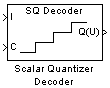# Scalar Quantizer Decoder

Convert each index value into quantized output value

## Library

Quantizers

`dspquant2`

•## Description

The Scalar Quantizer Decoder block transforms the zero-based input index values into quantized output values. The set of all possible quantized output values is defined by the Codebook values parameter.

Use the Codebook values parameter to specify a matrix containing all possible quantized output values. You can select how you want to enter the codebook values using the Source of codebook parameter. When you select `Specify via dialog`, type the codebook values into the block parameters dialog box. When you select `Input port`, port C appears on the block. The block uses the input to port C as the Codebook values parameter.

The input to this block is a vector of integer index values, where 0 ≤ index <N and N is the number of distinct codeword vectors in the codebook matrix. Use the Action for out of range index value parameter to determine what happens when an input index value is outside this range. When you want any index value less than 0 to be set to 0 and any index value greater than or equal to N to be set to N -1, select `Clip`. When you want to be warned when clipping occurs, select `Clip and warn`. When you want the simulation to stop and the block to display an error when the index values are out of range, select `Error`.

### Data Type Support

The data type of the index values input at port I can be `uint8`, `uint16`, `uint32`, `int8`, `int16`, or `int32`. The data type of the codebook values input at port C can be `double`, `single`, or Fixed-point.

The output of the block is the quantized output values. If, for the Source of codebook parameter, you select `Specify via dialog`, the Codebook and output data type parameter appears. You can use this parameter to specify the data type of the codebook and quantized output values. In this case, the data type of the output values can be `Same as input`, `double`, `single`, `Fixed-point`, or `User-defined`. If, for the Source of codebook parameter you select `Input port`, the quantized output values have the same data type as the codebook values input at port C.

## Dialog Box

The Main pane of the Scalar Quantizer Decoder block dialog appears as follows.Action for out of range index value

Use this parameter to determine the block's behavior when an input index value is out of range, where 0 ≤ index <N and N is the length of the codebook vector. Select `Clip`, when you want any index values less than 0 to be set to 0 and any index values greater than or equal to N to be set to N -1. Select `Clip and warn`, when you want to be warned when clipping occurs. Select `Error`, when you want the simulation to stop and the block to display an error when the index values are outside the range.

Source of codebook

Choose `Specify via dialog` to type the codebook values into the block parameters dialog box. Select ```Input port``` to specify the codebook using input port C.

Codebook values

Enter a vector of quantized output values that correspond to each index value. Tunable (Simulink).

The Data Types pane of the Scalar Quantizer Decoder block dialog appears as follows.Codebook and output data type

Specify the data type of the codebook and quantized output values. You can select one of the following:

• A rule that inherits a data type, for example, `Inherit: Same as input`.

• A built in data type, such as `double`

• An expression that evaluates to a valid data type, for example, `fixdt(1,16)`

Click the buttonto display the Data Type Assistant, which helps you set the Output data type parameter.

This parameter is available only when you set the Source of codebook parameter to ```Specify via dialog```. If you set the Source of codebook parameter to `Input port`, the output values have the same data type as the input codebook values.

## References

Gersho, A. and R. Gray. Vector Quantization and Signal Compression. Boston: Kluwer Academic Publishers, 1992.

## Supported Data Types

PortSupported Data Types

I

• 8-, 16-, and 32-bit signed integers

• 8-, 16-, and 32-bit unsigned integers

C

• Double-precision floating point

• Single-precision floating point

• Fixed point

• 8-, 16-, and 32-bit signed integers

Q(U)

• Double-precision floating point

• Single-precision floating point

• Fixed point

• 8-, 16-, and 32-bit signed integers

• 8-, 16-, and 32-bit unsigned integers

For more information on what data types are supported for each quantizer mode, see Data Type Support.

 Quantizer (Simulink) Simulink Scalar Quantizer Design DSP System Toolbox Scalar Quantizer Encoder DSP System Toolbox Uniform Encoder DSP System Toolbox Uniform Decoder DSP System Toolbox

## Extended Capabilities

### Fixed-Point ConversionDesign and simulate fixed-point systems using Fixed-Point Designer™.

Introduced before R2006a

Watch now